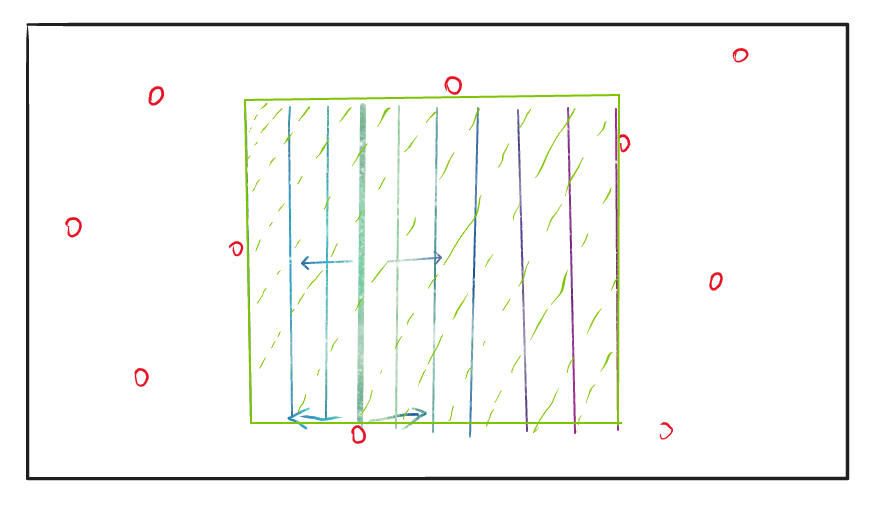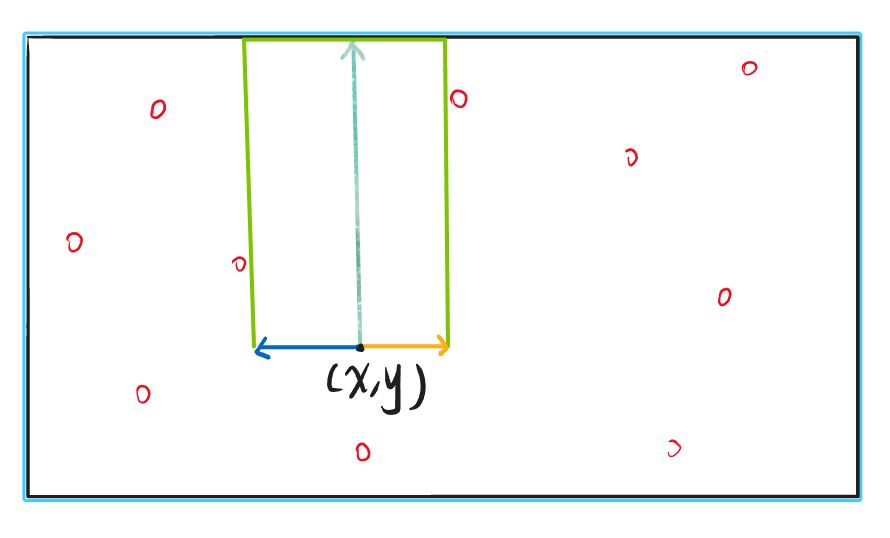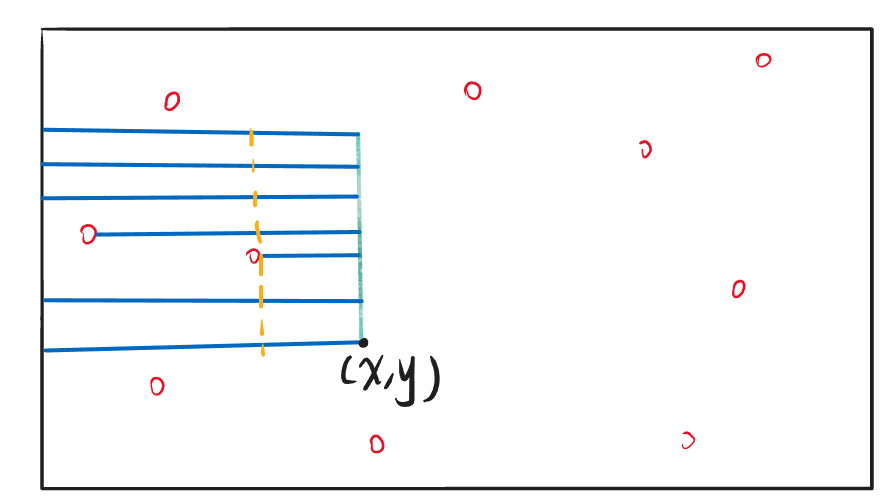## 悬线法思想及实现up[x][y] 为从$(x,y)$ 位置向上符合条件的最长线段长度。

L[x][y]为从$(x,y)$ 位置向左符合条件的最长线段长度。

R[x][y]为从$(x,y)$ 位置向右符合条件的最长线段长度。

up[x][y]=up[x-1][y]+1;
L[x][y]=L[x][y-1]+1;
R[x][y]=R[x][y+1]+1;$L[x][y]=min(L[x-1][y],L[x][y])$

$R[x][y]=min(R[x-1][y],R[x][y])$

for(int i=1;i<=n;i++){
for(int j=1;j<=m;j++){
if(i>=2 && a[i][j]与a[i-1][j]属于同一悬线){
L[i][j]=min(L[i-1][j],L[i][j]);
R[i][j]=min(R[i-1][j],R[i][j]);
}
}
}


1. 从该点除法向上延伸的悬线长度
2. 从该点位置向左，悬线能平移的最长距离
3. 从该点位置向右，悬线能平移的最长距离

## 模板例题

Q.E.D.

••( ﾉ^ω^)ﾉﾟ 稻 花 香 里 说 丰 年 ， 听 取 WA 声 一 片 。(╥╯^╰╥)